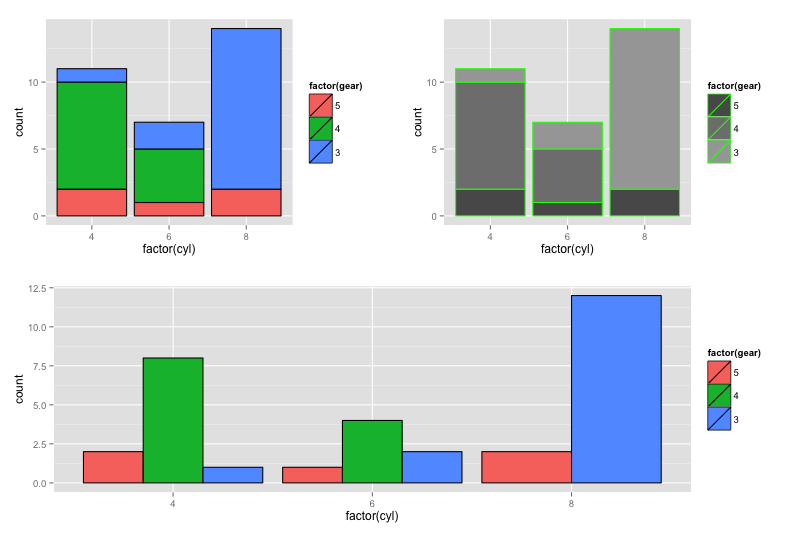# Multiple Plots (ggplot)

#### The multiplot() Function

Winston Chang’s R Graphical Cookbook provides a useful function to simplify the creation of layouts with multiple plots.  The function accepts ggplot objects as inputs.

Use of the function is straightforward.  First, multiplot() needs to be sourced and available in memory.  Then the plots need to be coded with variable assignments to create plot objects.  Finally, multiplot() is used to call the plot objects for placement in the predefined plot layout.